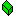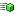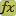Python3Script Xojo Plugin

#Python3Result Class (console safe)

A class that represents a result from a function call in Python 3.

Object
Python3Result

class Python3Result

#### ConstructorsPython3Result Constructs a empty Python3ResultPython3Result Constructs a result with Int32 valuePython3Result Constructs a result with Int64 valuePython3Result Constructs a result with UInt64 valuePython3Result Constructs a result with double valuePython3Result Constructs a result with string valuePython3Result Constructs a result with boolean valuePython3Result Constructs a result with object value

#### MethodsBooleanValue Returns boolean value from the resultBytesValue Returns Bytes value from the result as Python3BytesDictionaryValue Returns the internal Dictionary value as a Python3Dictionary instance. This is used to read keyed multiple results.DoubleValue Returns double value from the resultFitsInInt32 Returns true if the value at given index fits into 32 bit integer.shared FromBytes Constructs Python3Result instance from Bytes (Python3Bytes) instance.GetPtr Gets a pointer to the inner Python object of the Python3Result class.Int32Value Returns Int32 value from the resultInt64Value Returns Int64 value from the resultIsBoolean Returns true if the Result is BooleanIsBytes Returns true if the Result is BytesIsDictionary Returns true if the result is a DictionaryIsDouble Returns true if the Result is DoubleIsInteger Returns true if the parameter at given index is Integer. This means Integer from Python’s perspective. Which from Xojo’s perspective can be any kind of Integer. Python does not differentiate between them.IsObject Returns true if the Result is Xojo or Real Studio objectIsString Returns true if the Result is StringIsTuple Returns true if the result is a TupleObjectValue Returns a object field from the result. Object field here means that Xojo or Real Studio object gets stored in a Python scriptStringValue Returns string value from the resultTupleValue Returns the internal tuple value as a Python3Parameters instance. This is used to read multiple results.UInt64Value Returns UInt32 value from the result

#### Supported Platforms:

• MacOS X Cocoa 32 bit
• MacOS X Cocoa 64 bit
• Windows 32 bit
• Windows 64 bit
• Linux 32 bit
• Linux 64 bit
• Linux ARM